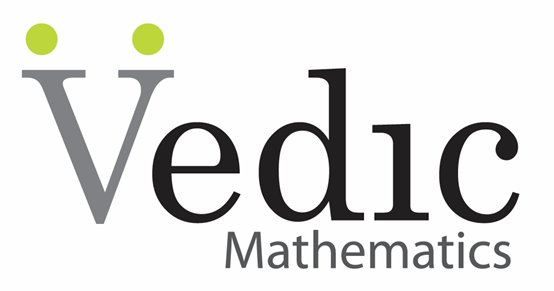CAT  >  Practice Problems: Vedic Mathematics

# Practice Problems: Vedic Mathematics - Notes | Study Quantitative Aptitude (Quant) - CAT

 1 Crore+ students have signed up on EduRev. Have you?Question 1: 46 × 44?
A. 2026
B. 2084
C. 2042
D. 2024

• To calculate 46 × 44,
• Multiply the unit’s digits = 6 × 4 = 24
• Then multiply 4, which is the tenth’s digit with 5 i.e. (1 + 4) = 20
• Therefore, the answer is 2024.

Question 2: Find the square of 89
A. 8021
B. 7821
C. 8121
D. 7921

• (89)2 = 82|2 × 8 × 9|92 = 81| (carry 14)4| (carry 8)1 = 7921

Question 3: Find the square of 107
A. 11449
B. 11249
C. 11439
D. 11349

• 107 is 7 more than the base 100, 72 is 49. Then the left side is 107 + 7 = 114. Thus, the square becomes 11449

Question 4: 43 × 34
A. 1562
B. 1262
C. 1462
D. 1264

• 43 × 34 = 4 × 3|4 × 4 + 3 × 3|3 × 4
= 12| (carry 2)5| (carry 1) 2 = 1462

Question 5: 37 × 73
A. 2901
B. 2801
C. 2701
D. 2781

• 37 × 73 = 3 × 7|3 × 3 + 7 × 7|7 × 3
= 21| (carry 5)8| (Carry 2)1 = 2701

Question 6: 82 × 71
A. 5922
B. 5022
C. 5822
D. 1422

82 x 71 is calculated as:

• Step 1: 2 × 1 = 2 (Write 2 as last digit)
• Step 2: 1 × 8 + 2 × 7 = 22 (Write 2 and 2 is carried over to the next step)
• Step 3: 8 × 7= 56. Therefore, 5822 is the correct answer.

Question 7: 976 × 653
A. 637328
B. 647358
C. 876458
D. 983458

976 × 653 is calculated as:

• Step 1: 6 × 3 = 18 (Write 8 as the last digit and 1 is carried over to the next step)
• Step 2: 7 × 3 + 6 × 5 + 1(Carried Over) = 52 (Write 2 and 5 is carried over to the next step)
• Step 3: 9 × 3 + 6 × 6 + 7 × 5 + 5 (Carried Over) = 98 (Write 8 and 9 is carried over to the next step)
• Step 4: 9 × 5 + 7 × 6 + 9(Carried Over) = 96 (Write 6 and 9 is carried over to the next step)
• Step 5: 9 × 6 + 9 (Carried Over) = 63 (Write 39). Therefore, 637328 is the answer.

Question 8: 107 × 93
A. 8971
B. 9951
C. 9451
D. 7581

107 x 93 is calculated as:

• Step 1: 107 -100 = 7, 93-100= -7
• Step 2: 7 × -7 = -49, 100 -49 =51(last two digits)
• Step 3: 107-7= 93+7 = 100 -1 (borrowed) =99
• Therefore, the answer is 9951

Question 9: 109 × 96
A. 10264
B. 10464
C. 10864
D. 10364

109 X 96 is calculated as:

• Step 1: 109 -100 = 9, 96-100= -4
• Step 2: 9 × -4 = -36, 100 -36 =64(last two digits)
• Step 3: 109-4= 96+9 = 105 -1 (borrowed) =104
• Therefore, the answer is 10464.

Question 10: Find the square of 97
A. 8109
B. 9809
C. 9409
D. 9209

• 97 is 3 less than the base 100. While squaring 97, the right side will be (3)2 i.e. 09. And the left side would be the ‘number given – difference’ i.e. 97 – 3 = 94.
• So, the square of 97 is 9409.
The document Practice Problems: Vedic Mathematics - Notes | Study Quantitative Aptitude (Quant) - CAT is a part of the CAT Course Quantitative Aptitude (Quant).
All you need of CAT at this link: CAT

## Quantitative Aptitude (Quant)

163 videos|163 docs|131 tests
 Use Code STAYHOME200 and get INR 200 additional OFF

## Quantitative Aptitude (Quant)

163 videos|163 docs|131 tests

### How to Prepare for CAT

Read our guide to prepare for CAT which is created by Toppers & the best Teachers

Track your progress, build streaks, highlight & save important lessons and more!

,

,

,

,

,

,

,

,

,

,

,

,

,

,

,

,

,

,

,

,

,

;